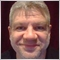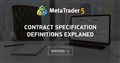# How to calculate correct LOT size for NAS100 - page 248

Alain Verleyen:
The leverage has nothing to do with this calculation.
Thanks for that bit of info.48

Alain Verleyen:

You don't need to divide by 10, from where this 10 comes from ?

Supposing the account is USD and you are using standard lot (100,000), the correct answer is 27.5 lots.

The correct formula is :

lot = capital to risk / (sl"in ticks"*tickValue).

On Forex, usually 1 tick = 1 point (0.00001 for a 5 digits pair).

The tick value = contract size * tick size * conversion rate (from profit currency to account currency).

tick value is provided by mql code but as Keith noticed, sometimes the value provided by the broker is not correct, you can calculate it yourself.

But I still don't know how to calculate that NAS100 lot size.42817

Stephan:

But I still don't know how to calculate that NAS100 lot size.

What is so hard to apply a simple formula ?

lot = capital to risk / (sl"in ticks"*tickValue).48

Alain Verleyen:

What is so hard to apply a simple formula ?

Because I don't know the tickValue of NAS100. That is the whole point of this thread.

8895.30? What is the value of that?42817

Stephan:

Because I don't know the tickValue of NAS100. That is the whole point of this thread.

8895.30? What is the value of that?

The tick value = contract size * tick size * conversion rate (from profit currency to account currency).

tick value is provided by mql code but as Keith noticed, sometimes the value provided by the broker is not correct, you can calculate it yourself.Moderator
32953

When you refer to pips for non-forex instruments, you should always state what you consider to be a pip.

For forex instruments, there are accepted pip values, not necessarily so for indexes, commodities etc.

With an index such as the Nasdaq, I believe that the contract size is 1, but this should always be checked for the broker that you are using.

If the current price of the Nasdaq is \$8930.00 and the contract size is 1, then every 0.01 movement is \$0.01, every 1.00 movement is \$1 (for 1 lot)

Example. I want to open a sell order on NAS100. I have \$50000 in my account. I want to risk 10% (\$10000). My stoploss is 200 pips.

Let's say that you consider a pip to be 0.1, so pip value for 1 lot is 10 cents. (this will need to be converted if the account currency is not USD)

A loss of 200 pips with 1 lot will be 200*10 cents=\$20

You are risking \$10,000 so the lot size will be 10,000/20=500

Now the calculation may not always result with a nice even lot size, so it must be checked that it is at least the minimum lot size and not more than the maximum allowed by the broker. It should also be normalised to lot step. ie. if the calculated lot size is 1.15 and the lot step is 0.1, you must round it up to 1.2 or down to 1.1

You can find these values by right clicking on the symbol in the market watch window and clicking specifications. Or view Symbols list and find the symbol and double clicking it.48

Keith Watford:

For forex instruments, there are accepted pip values, not necessarily so for indexes, commodities etc.

Thanks for that info.

With an index such as the Nasdaq, I believe that the contract size is 1, but this should always be checked for the broker that you are using.

I believe I found the contract size to be \$20.

Let's say that you consider a pip to be 0.1, so pip value for 1 lot is 10 cents. (this will need to be converted if the account currency is not USD)

I'll start referring to ticks for NAS100 :) (All the training I did was for forex where a pip = 0.0001 (Except for JPY))

You can find these values by right clicking on the symbol in the market watch window and clicking specifications. Or view Symbols list and find the symbol and double clicking it.

Jip. That was where I found it.

Thanks for all the answers. I'll adjust my formulas accordingly and give it a go.Moderator
32953

Stephan:

I believe I found the contract size to be \$20.

I should double check that if I were you.

I am not aware of any broker that has 1 contract to be any thing other than the unit cost.

If it is, then my calculations will not be any good.48

Keith Watford:

When you refer to pips for non-forex instruments, you should always state what you consider to be a pip.

For forex instruments, there are accepted pip values, not necessarily so for indexes, commodities etc.

With an index such as the Nasdaq, I believe that the contract size is 1, but this should always be checked for the broker that you are using.

If the current price of the Nasdaq is \$8930.00 and the contract size is 1, then every 0.01 movement is \$0.01, every 1.00 movement is \$1 (for 1 lot)

Example. I want to open a sell order on NAS100. I have \$50000 in my account. I want to risk 10% (\$10000). My stoploss is 200 pips.

Let's say that you consider a pip to be 0.1, so pip value for 1 lot is 10 cents. (this will need to be converted if the account currency is not USD)

A loss of 200 pips with 1 lot will be 200*10 cents=\$20

You are risking \$10,000 so the lot size will be 10,000/20=500

Now the calculation may not always result with a nice even lot size, so it must be checked that it is at least the minimum lot size and not more than the maximum allowed by the broker. It should also be normalised to lot step. ie. if the calculated lot size is 1.15 and the lot step is 0.1, you must round it up to 1.2 or down to 1.1

You can find these values by right clicking on the symbol in the market watch window and clicking specifications. Or view Symbols list and find the symbol and double clicking it.

Hi

I made a couple of spot trades to confirm. Maths work 100%.

But what I saw now is the trade volume limit is 2. That's a far cry from 500.

I took a look at another broker I tried previously, and compared the two contract specifications. Big differences.

I started a new thread here https://www.mql5.com/en/forum/330098. Hoping someone can help explain some of those specifications for me like "Stops Level", "Margin Maintenance" and "Margin Percentage"...

Thanks again!Contract Specification definitions explaned
• 2020.01.09
• www.mql5.com
Hi I'm hoping someone can help my to explain what all these contract specifications mean...48

Keith Watford:

I should double check that if I were you.

I am not aware of any broker that has 1 contract to be any thing other than the unit cost.

If it is, then my calculations will not be any good.

Hi

At the broker I'm currently using, AxiTrader, the contract size is \$20.

At JP Markets the contract size is \$100.

I'm trying to add a screenshot to this post but can't seem to.